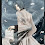## Wednesday, May 28, 2014

### New version of plotTree.wBars that permits positive & negative phenotypic trait values

A couple of weeks ago I posted a new function (1, 2) to plot bars at the tips of a circular or square phylogram. One limitation of this function is that because the bars are plotted 'growing' out of each leaf of the tree, the values of the phenotypic trait data underlying the bars cannot be negative. Negative values would result in bars growing (in the case of a fan tree) towards the root of the tree - which, of course, does not look right at all.

Here's what I mean:

> tree<-pbtree(n=100)
> x<-fastBM(tree)
> plotTree.wBars(tree,x,scale=0.5)
> ## or setting type="fan"
> plotTree.wBars(tree,x,scale=0.5,type="fan")

Now let's try the new version:

> require(phytools)
> packageVersion("phytools")
 ‘0.4.11’
> plotTree.wBars(tree,x,scale=0.5)
> plotTree.wBars(tree,x,scale=0.5,type="fan")

I'm not sure it makes a great visual in this case - but, nonetheless, it's better.

When some values of x are negative and the tree is non-ultrametric, then the bars are also centered a constant distance from the maximum tip height in the tree. For instance:

> tree<-rtree(n=50)
> x<-fastBM(tree)
> plotTree.wBars(tree,x,scale=0.3)

The code for this new function version is here; but you can also install a new build of phytools from source with this update.

1.NIce function! I wonder can we replace the bar plot with an arcplot? For example, we have a list of species, and we know their evolutionary distance and their interactions (e.g. spA co-occurrent with spB; spB co-occurrent with spC, or any other kind of interaction.). We can plot their interaction network as an arcplot. At the same time, we also can plot their phylogeny in the same plot. (https://github.com/gastonstat/arcdiagram/issues/3)

I figured out how to plot the arcplot with this package (https://github.com/gastonstat/arcdiagram). But since I am not familiar with either these two packages (ape and arcdiagram), I do not know how to plot the phylogeny and the arcplot in the same window. I can use `par(mfrow = c(1,2))` but these two plots will not align well.

I read the source code of the arcdiagram package and it is not a complex one. But the ape and your phytools are. As a result, I think the fastest way is just comment here and ask for your help.

Any idea/interests to put this thought into a function?
Thanks.
Daijiang

1.Sure. Can you send me an example of what this should look like & a sample dataset. Email me at liam.revell@umb.edu. - Liam

2.Sir, I am sorry but when I run the commands I get
> require(phytools)
Warning message:
package ‘phytools’ was built under R version 3.1.0
> tree<-pbtree(n=100)
> x<-fastBM(tree)
> plotTree.wBars(tree,x,scale=0.5)
Error: could not find function "plotTree.wBars"

3.Yes it works after the new function is run, thanks

1.This comment has been removed by the author.

4.Hi!
I am really interested in using this script but I had some issues running the function on my data... I have a tree, newick format, and one vector of numerical phenotypic data (a text file, tab delimited, 2 columns: names corresponding to the leaves of the tree and the phenotypic values, imported as a table with header=TRUE and row.names=1).
When I try to run :
plotTree.wBars(tre, traits, type="fan", scale=1)
I obtain:
Error in if (min(x) < 0) h <- max(nodeHeights(tree)) :
missing value where TRUE/FALSE needed

Any idea of what went wrong...?

Thanks!!

Jonathan

1.Hi Jonathan.

I believe that x should be a vector with names, not a table or matrix.

Try:

x<-setNames(traits[,1],rownames(traits))
plotTree.wBars(tre,x,type="fan",scale=1)

Let us know if that works.

- Liam

5.Hi Liam,
It seems to work! thanks!!! I might have a problem of scale though: bars are huge compare to the tree... But thanks for fixing my issue!!!!!

6.Hi Liam,
works perfectly, thanks.
Another quick question, is it possible to print tip labels? I tried the option show.tip.label = TRUE but did not seem to work...
Thanks again!

7.Hi again Liam...
Using a different dataset, I have the same error message even after using the "setNames" trick...
Phylogeny is newick format (109 tips), trait is numeric (imported with read.table with row.names=1, then transformed with "setNames")...
No null branch, no missing phenotypic data...
The error is exactly the same:

Error in if (min(x) < 0) h <- max(nodeHeights(tree)) :
missing value where TRUE/FALSE needed

Is the problem could come from a different issue...?

thanks so much!

Jonathan

1.Hi Jonathan.

Not sure. Try saving your R workspace & emailing it to me. I will troubleshoot.

- Liam

2.Hi Liam,
Just to let you know that I figured out where the issue was... It was as silly as a capital letter in the data file for a species name vs lowercase letter in the tree file...
It all works perfectly now...
Sorry for the confusion!
Best,
Jo

3.Hey. I'm glad to hear that you resolved it. Sorry I didn't get to it first. - Liam

8.This comment has been removed by the author.

9.This comment has been removed by the author.

10.Hi Liam,
Is there a way to color the bars by a categorical trait value? I have only been able to figure out how to color branches by a trait, but not the tip labels or bars. In your first couple example figures, if you wanted to color bars differentially based on State A or B, how would you do that?
Thanks!
-Katie

1.Hi Katie.

In the function version plotTree.barplot this is possible. Here is an example.

- Liam

11.Hi Liam,
I'm encountering a little bump trying to use this function and I can't figure out what's wrong:

> plotTree.wBars(tree=tr, x=dat)
Error in plot.window(xlim = xlim, ylim = ylim, asp = asp) :
need finite 'xlim' values

Including an xlim argument doesn't work:
> plotTree.wBars(tree=tr, x=dat, xlim=c(0,100), ylim=c(0,100))
Error in plot.window(xlim = xlim, ylim = ylim, asp = asp) :
need finite 'xlim' values

Thanks for any help!

Note: due to the very large amount of spam, all comments are now automatically submitted for moderation.